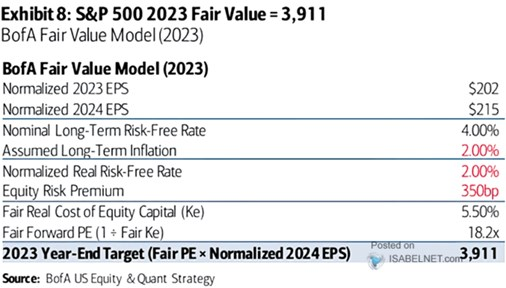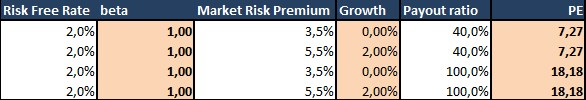# Fair value of US stocks according to Bank of America. Something for valuation connoisseurs

--

came up with an estimate of the fair value of the US stock market and also shows the basic inputs that go into its estimate. Let’s take a look today at how this bank’s analyst team thinks. It’s quite for valuation connoisseurs.

The following table shows that in the described scenario for this year, BofA calculates profits in the SPX index at 202 dollars per share (so a noticeable profit recession). And for next year at \$215 per share. For comparison, he sees \$224 per share for this year (approx. year-on-year stagnation) and \$247 for 2024. The logic used in BofA is then simple – to put a “fair” PE derived from the fundamental on the estimated profits for 2024.1. Risk-free rates: The fair PE bank estimates from real values ​​that risk-free rates at the nominal level will reach 4%, inflation is 2%. Therefore, real rates reach 2% and this corresponds to the real growth of the economy. I wouldn’t fault such a view, it sticks to estimates of the potential of the US economy and that revenues could equal growth.

2. Risk premium: Fair PE includes not only risk-free rates, but the required return on stocks given these rates and risk premiums. And here BofA is operating with a number that I would see as very low considering historical experience and the current context. According to BofA, the risk premium of the US market is 3.5%. Which is somewhere on the low end of historical values ​​(for the studies I’ve seen).

Perhaps BofA estimates historical risk premiums by subtracting risk-free returns from the inverted PE. But the result, despite the claims of many, is not a market risk premium, but a premium mixed with the expected growth of G and the POR payout ratio. I will come back to this further below, for now I will just point out that I work with a risk premium of 5.5% based on studies dealing with this topic. And my real cost of equity/required return would then come out not to 5.5% (BofA’s number as 3.5% premium and 2% real rate) but to 7.5%. Nominal at 9.5%.

3. Fair PE: At the end of the table, BofA then makes a calculation that is difficult for me to understand, where the PE is calculated as 1 divided by the real cost of capital. Mr. Gordon once helped us with similar considerations, who showed that the value of a stock is equal to: Current dividends D divided by required return R minus dividend growth G.

In the PE calculation from BofA, D (respectively the ratio of profits and dividends POR) and G are missing. For example, if companies on the market paid out 40% of their profits in dividends, the numerator would not have a value of 1, but 0.4. And the denominator would not be R, but R – G. And the PE result would not be 18.2, but 11.3. If I then replaced the risk premium and calculated “my” 5.5% instead of 3.5%, the derived fair PE would be 7.2

4. How to put it together? As I write in point 2, BofA calculates the market risk premium as the difference between the inverse PE and the risk-free rates. However, the aforementioned G and POR (their historical values) are also implicitly reflected in this number. It occurs to me that the bank calls this number the risk premium of the market, but then uses it in the PE calculation knowing that it also reflects G and POR. Therefore, PE optically calculates “only” from the required return, but actually from all variables, because the cost of equity in its version includes all the necessary variables. But I am afraid that the construction of the formula then makes it only an approximation. And of course the nomenclature doesn’t fit.

5. Total sum: With what the bank estimates for profits and what the bank estimates for the calculation of fair PE and how it deals with it, it comes out that the fair value of the SPX index at the end of the year is 3911 points. Evidently, this is a scenario with a noticeable profitable recession, when profits will not reach the levels of 2022 even next year. However, the used PE around 18+ in such a scenario does not fit my intuition and historical experience with development during a recession. If I then assume that the risk premium given by the bank is just a risk premium and add other necessary variables (G and POR), I get a significantly lower PE (and consequently the value of the index).

As I warned at the beginning, the above is more for those who are interested in a more detailed account of the valuation of the stock market, or rather the approach chosen at BofA.*Just as a footnote, I’ll show that we get to BofA’s resulting PE if we use its risk premium and no earnings/dividend growth, or a 5.5% premium and 2% expected dividend growth. In both cases, however, we have to count on the fact that companies will pay out all of their profits in dividends (100% POR). Historically, even the 40% used in the alternative calculation is enough.

The article is in Czech

-